Abstracts

In this paper are determined the principal curvatures and principal curvature lines on canal surfaces which are the envelopes of families of spheres with variable radius and centers moving along a closed regular curve in R³. By means of a connection of the differential equations for these curvature lines and real Riccati equations, it is established that canal surfaces have at most two isolated periodic principal lines. Examples of canal surfaces with two simple and one double periodic principal lines are given.

Riccati equation; principal curvature lines; canal surfaces

Neste trabalho são determinadas as curvaturas principais e as linhas de curvatura principal das superfícies canal que são as envoltórias de famílias de esferas com raios variáveis e centros deslocando-se ao longo de curvas fechadas regulares em R³. A partir de uma conexão com as equações de Riccati mostra-se que estas superfícies têm no máximo duas curvas principais periódicas isoladas. São dados exemplos com duas curvas principais periódicas simples e com uma dupla.

Equação de Riccati; linha de curvatura principal; superfície canal

MATHEMATICAL SCIENCES

Lines of principal curvature on canal surfaces in R3

Ronaldo GarciaI; Jaume LlibreII; Jorge SotomayorIII

IInstituto de Matemática e Estatística, Universidade Federal de Goiás - Cx. Postal 131, 74001-970 Goiânia, GO, Brasil

IIDepartament de Matemàtiques, Universitat Autònoma de Barcelona - 08193 Bellaterra, Barcelona, Spain

IIIInstituto de Matemática e Estatística, Universidade de São Paulo - Rua do Matão, 1010, Cidade Universitária, 05508-090 São Paulo, SP, Brasil

ABSTRACT

In this paper are determined the principal curvatures and principal curvature lines on canal surfaces which are the envelopes of families of spheres with variable radius and centers moving along a closed regular curve in R3. By means of a connection of the differential equations for these curvature lines and real Riccati equations, it is established that canal surfaces have at most two isolated periodic principal lines. Examples of canal surfaces with two simple and one double periodic principal lines are given.

Key words: Riccati equation, principal curvature lines, canal surfaces.

RESUMO

Neste trabalho são determinadas as curvaturas principais e as linhas de curvatura principal das superfícies canal que são as envoltórias de famílias de esferas com raios variáveis e centros deslocando-se ao longo de curvas fechadas regulares em R3. A partir de uma conexão com as equações de Riccati mostra-se que estas superfícies têm no máximo duas curvas principais periódicas isoladas. São dados exemplos com duas curvas principais periódicas simples e com uma dupla.

Palavras-chave: Equação de Riccati, linha de curvatura principal, superfície canal.

1. INTRODUCTION

The study of principal curvature lines, along which a surface in3 bends extremely and their umbilic singularities was founded by Monge, Dupin and Darboux. See (Gray 1998) and (Sotomayor 2003) for references. For the basic facts about principal curvature lines on surfaces the reader is addressed to (do Carmo 1976), (Spivak 1979) and (Struik 1988).

As a consequence of the work of Monge and Dupin the lines of curvature on quadrics and toroidal - Dupin Cyclides - surfaces were determined. See (Fischer 1986, Chap. 3), for an outline of the theory and for a collection of remarkable illustrations.

In (Gutierrez and Sotomayor 1982, 1991, 1998) ideas originating in the Qualitative Theory of Differential Equations and Dynamical Systems, such as Structural Stability and Genericity, were incorporated into the subject; see also (Garcia and Sotomayor 2002). Historical comments, going up to some recent developments on principal curvature lines, can be found in (Sotomayor 2003).

The global dynamic complexity of principal curvature in simple smooth surfaces was illustrated in (Gutierrez and Sotomayor 1991, 1998). This includes examples of recurrent (dense) principal curvature lines on spheroidal surfaces, which are small perturbations of ellipsoids of revolution and canal surfaces with constant radial functions. The methods established in these works show how to make hyperbolic a principal cycle by means of a small smooth perturbation. This leads to smooth toroidal immersions with arbitrary large number of principal isolated principal cycles.

In this paper is improved and reproved a result that goes back to Vessiot, establishing that principal curvature lines on canal surfaces immersed in3 (see Section 2, Definition 2 verify a Riccati equation (see Section 3, Remark 7). In fact, (Vessiot 1919) is the first source known by the authors for the connection between Riccati equations and principal curvature lines on canal surfaces. The improvement consists in the formulation of precise conditions for canal surfaces be regular immersions and also to have umbilic points (see Section 3, Theorem 4 and Remark 5). A consequence of the Riccati structure for principal curvature lines on canal immersed surfaces implies that the maximal number of isolated periodic principal lines is 2. Examples of canal surfaces with two (simple i.e. hyperbolic) and one (double i.e. semi-stable) principal periodic lines, absent in (Vessiot 1919) and also in later references, are given in this paper (see Section 4, Proposition 8).

2. REGULAR CANAL SURFACES

Consider the space3 endowed with the Euclidean inner product < , > and norm | | = < , > 1/2 as well as with a canonical orientation. The wedge product Ù of vectors is defined relative to this orientation.

Let c be a smooth regular closed curve immersed in3, parametrized by arc length s Î [0, L]. This means thatAssume also that the curve is bi-regular. That is:Along c is defined its moving Frenet frame {t,n,b}. Following (Spivak 1979) and (Struik 1988), this frame is positive, orthonormal and verifies Frenet equations:Equations (1) to (3) define the unit tangent, t, principal normal, n, curvature, k, binormal, b = t Ù n, and torsion, t, of the immersed curve c.

PROPOSITION 1. Let r(s) > 0 and q(s) Î ]0, p[ be smooth functions of period L. The mapping a :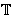2 =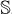1 ×1®3 , defined on2 modulo L × 2p byis tangent to the sphere of center c(s) and radius r(s) if and only if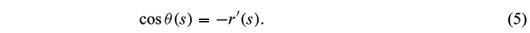Assuming (5), with r' (s) < 1, a is an immersion provided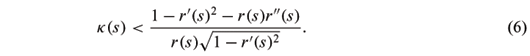PROOF. Calculations using equations (3) give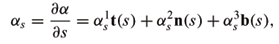whereHere, of course, r, q, t, k are functions of s. Also,The unit normal vector pointing inward the sphere |p - c(s)| = r(s) with center c(s) and radius r(s) at p = a(s, j) is Na = ( c(s) - a(s, j))/r(s), which is given by:Clearly áaj, Nañ = 0. Calculation gives áas, Nañ = -(cosq + r') . Therefore, the condition of tangency at (s, j) of a to the sphere |p - c(s)| = r(s) is cosq(s) = -r'(s).At this point it is appropriate to write the mapping a and its derivatives involving only functions of r(s) rather than of q(s), replacing the expressions cosq(s) = -r'(s), sinq(s) = (1 - (r')2)1/2 in equations (4) and (7).

The expression for E is as follows:Additional calculation and simplification using equations (9), with the tangency condition (5) imposed, and (10) gives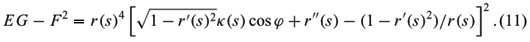This expression, which is equal to |¶a/¶s Ù ¶a/¶j|2, vanishes if and only ifCondition (6) states that the absolute value of the right-hand member of (12) is larger than 1, which by (11) implies the linear independence of as and aj at every point (s, j), namely that a is an immersion.

DEFINITION 2. A mapping such as a, of2 into3, satisfying conditions (5) and (6) will be called an immersed canal surface with center along c(s) and radial function r(s). When r is constant, it is called an immersed tube. Due to the tangency condition (5), the immersed canal surface a is the envelope of the family of spheres of radius r(s) whose centers range along the curve c(s).

REMARK 3. Proposition 1 is partially found in (Vessiot 1919). There, however, the regularity condition (6) was overlooked. Other references for canal surfaces are (Blaschke 1929) and (Gray 1998).

3. PRINCIPAL LINES ON REGULAR CANAL SURFACES

In this work the positive orientation on the torus2 =1 ×1 is defined by the ordered tangent frame {¶/¶s ,¶/¶j}. Therefore the positive unit normal -or Gaussian map- Na of the immersion a is defined by |¶a/¶s Ù ¶a/¶j|Na = ¶a/¶s Ù ¶a/¶j. By the tangency condition (5) this unit vector is given by (8), which can be written as follows:It points inwards the toroidal surface defined by a.

Below will be studied the global behavior of the principal curvature lines of a.

THEOREM 4. Let a :1 ×1®3 be a smooth immersion expressed by (4). Assume the regularity conditions (5) and (6) as in Proposition 1 and also that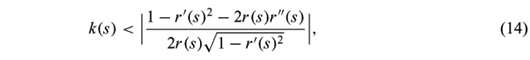The maximal principal curvature lines are the circles tangent to ¶/¶j. The maximal principal curvature is

k2(s) = 1/r(s)

The minimal principal curvature lines are the curves tangent toThe expressionis negative, and the minimal principal curvature is given by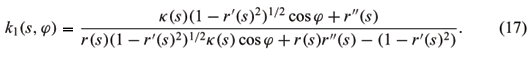There are no umbilic points for a: k1(s,j) < k2(s).

PROOF. Direct calculation gives:By Rodrigues equation, see (do Carmo 1976) and (Struik 1988), the circles s = constant are principal curvature lines of a, with principal curvature k2(s).

Denoting the metric of a by Eds2 + 2Fdsdj + Gdj2, it follows that the direction orthogonal to ¶/¶j, giving the other principal direction, is defined by the vector fieldwhich by equation (9) is collinear with V(s, j) in (15).

Differentiation of (13) using Frenet equations (3) givesandSubstitution, taking into account equation (15), leads to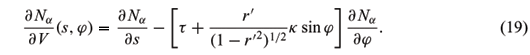Similarly for a, calculation of its derivative ¶a/¶V, givesFurther calculation using equations (18), (19), (20) and the expression for k1(s, j) given in equation (17), follows thatThis is Rodrigues equation, which establishes that, in fact, k1 is a principal curvature.

From (6) it follows that (16), the denominator of k1, is always negative. This implies that k1 < 1/r(s) = k2. Otherwise, k1> k2 and, after direct manipulation, by (14) this would lead toThis prevents the existence of umbilic points and justifies the names maximal, for subscript 2, and minimal, for subscript 1, given in the statement.REMARK 5 [Umbilic Points in Canal Immersions]. The calculation of k1 and the condition for the appearance of umbilic points for regular canal surfaces has not been considered in previous works on the subject. The discussion leading to (21) leads to the following equation for umbilic points:It gives a non-empty curve if condition (14) is not imposed. This curve however consists of removable singularities for the principal line fields which have smooth extensions to the whole torus, given by ¶/¶j and V. Under generic conditions on the radial function, r(s), and curvature, k(s), functions it will be expected to appear curves of umbilic points as those studied in Proposition 2 of (Garcia and Sotomayor 2005).

REMARK 6 [Minimal Principal Foliation in terms of Differential Forms]. Written as a differential form, the vector field (15) becomes:When another parameter t is used, say T-periodic, related with the arc length s of c in the form s = s(t), with, the form in (22) can be written as:REMARK 7 [Vessiot]. By the change of coordinates tan(j/2) = z, equation (22) is transformed into the L-periodic Riccati equationTherefore, the solutions of equation (15) can be obtained from the solutions of equation (22) contained in [0, T] × [-p, p].

4. CANAL SURFACES WITH ONE AND TWO PRINCIPAL CYCLES

In this section is carried out a discussion on the qualitative properties of equation (15), or of its equivalent form (23), absent in (Vessiot 1919).

Being equivalent to a periodic Riccati equation, this equation has a Möebius transformation as return map (see Hille 1976) and therefore can have either:

(a) all its solutions periodic,

(b) all its solutions dense,

(c) two hyperbolic (simple) periodic solutions or

(d) one semi-hyperbolic (double or semi-stable) periodic solution.

An example of situation (a) is exhibited by the standard torus of revolution. An example of(b) is given in (Gutierrez and Sotomayor 1991, 1998), for a canal surface of constant radius(a tube) around a curve that is not bi-regular. Below will be given examples of cases (c) and (d). This will also provide examples of case (b) for bi-regular curves. The cost of this is heavier calculation which, nevertheless, is easy to corroborate with Computer Algebra.

The example consists in a deformation of the T = 2p-periodic plane elliptic curve

c(t) = (2cos t, sin t, 0),

whose curvature k(t) = k(t, 0) is k(t) = 2/(4 - 3cos2 t)3/2.

PROPOSITION 8. Consider the three parameter family of canal surfaces Se, r, µ around the curve ce(t) = (2cos t, sin t, e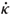(t)), with radial function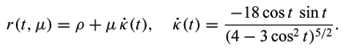There are two smooth curves e = e1(µ) = -µ + O12) and e = e2 (µ) = µ + O22),such that for any r, µ small and positive, the canal surface Se, r, µ has two hyperbolic principal cycles for e Î]e1(µ), e2(µ)[ and has one double principal cycle along the curves e = e1(µ) and e = e2(µ).

PROOF. Standard calculation gives:for the element of arc length of ce.

Also, the curvature of ce isHere will be needed only(t,0) =(t) as given above and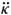(t) in the integralThe torsion of ce isHere will only be needed that the expressions for t(t, 0) = 0 and t1(t) = ¶t/¶e(t, 0).

From direct calculations follows thatThe differential equation corresponding to (23) for the 2p-periodic canal surface Se, r, µ is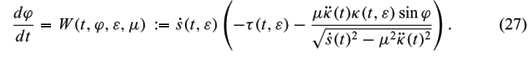Denote by P(j0, e, µ) the return map for equation (27). It is given by P(j0, e, µ) = F(2p, j0, e, µ), where F(t, j0, e, µ) is the solution of (27) such that F(0, j0, e, µ) = j0. Therefore, the 2p-periodic solutions are given by the implicit surface P(j0, e, µ) - j0 = 0. Since P(j0, 0, 0) = j0, the Fundamental Theorem of Calculus (Hadamard's Formula) implies thatWriteThe expressions for the derivatives of the solutions of the differential equations with respect to parameters applied to (27), which in the present case, following classical differential equations results, are the integrals of the non-homogeneous linear - called variational - equations. According to (Sotomayor 1979, page 42), (Chicone 1999, page 337) or (Coddington and Levinson 1955, page 30), where these classical results are proved, these equations and their initial conditions are as follows: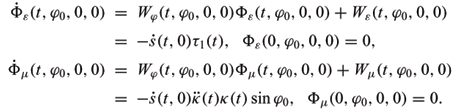Integrating these equations, taking into account equations (25) and (26), leads to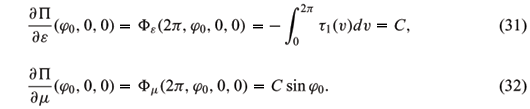Writing e = nm and substituting into (28), taking into consideration the expressions (31) and (32), we obtain

P(j0, e, µ) - j0 = Cµ(n + sin j0 + nZ(n,µ, j0)).

Therefore, the surface of 2p-periodic orbits, in the (n, m, j)-space consists of a plane µ = 0crossing transversally a regular sheet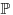at the sinusoidal curve of equation n + sin j0 = 0. The critical values of the projection on the (n, µ)-plane of the surface, coming from double (semi-stable) periodic solutions, the fold curve, must cross transversally the n-axis at points corresponding to n = ±1, which are the critical values of the projection of the sinusoidal curve. Going back to the coordinates (e, µ) gives the conclusion formulated in the proposition.REMARK 9 [Arnold Tongues]. Due to equations (31) and (27), for r small, the return map on the tube Se, r, 0 is a rotation with its rotation number changing monotonically with e (taken small). Therefore, it takes irrational values and the tube presents case (b). It also takes rational values, q/p, which are the vertices of the Arnold Tongues (in the (e, µ)-plane), corresponding to canal surfaces with periodic closed principal lines winding p-times around the parallels (t-circles) and q-times around the meridians (j-circles) of the torus. The sector with vertex at (0, 0) established in Proposition 8 is the principal tongue, with rotation number 0. See (Chicone 1999, page 372).

ACKNOWLEDGMENTS

The first and third authors are fellows of Conselho Nacional de Desenvolvimento Científico e Tecnológico (CNPq) and are partially supported by CNPq Grants Programa de Apoio ao Desenvolvimento Científico e Tecnológico (PADCT) 620029/2004-8 and 47.3824/2004-3. The second author is partially supported by La Dirección General de Investigación Ciencia y Técnica (DGICYT) grant number MTM2005-06098-C02-01 and by Centro de Investigación Científica y Tecnológica (CICYT) grant number 201SGR00173. The first and second authors are also partially supported by the joint project Coordenação de Aperfeiçoamento de Pessoal de Nível Superior - Ministério da Educação, Cultura e Desporto (CAPES-MECD) grants 071/04 and HBP2003-0017, respectively.

Manuscript received on October 10, 2005; accepted for publication on May 3, 2006; contributed by JORGE SOTOMAYOR*

AMS Classification (2000): 53C12, 34D30, 53A05, 37C75.

* Member Academia Brasileira de Ciências

Correspondence to: Ronaldo Garcia

E-mail: ragarcia@mat.ufg.br

• BLASCHKE W. 1929. Differential Geometrie, III, Differentialgeomtrie der Kreise und Kugeln, Springer-Verlag, Berlin.
• CHICONE C. 1999. Ordinary Differential Equations with Applications, Springer-Verlag, New York.
• CODDINGTON E AND LEVINSON N. 1955. Theory of Ordinary Differential Equations, Mc-Graw-Hill, New York.
• DO CARMO M. 1976. Differential Geometry of Curves and Surfaces, Prentice-Hall, Englewood-Cliffs.
• FISCHER G. 1986. Mathematical Models, Friedr. Vieweg & Sohn, Braunschweig.
• GRAY A. 1998. Modern Differential Geometry of Curves and Surfaces with Mathematica, Second Edition, CRC Press, New York, 1998.
• GARCIA R AND SOTOMAYOR J. 2002. Lecture Notes on Differential Equations of Classical Geometry, Preprint of Lecture Course delivered in Săo Carlos, SP, Brazil.
• GARCIA R AND SOTOMAYOR J. 2005. On the patterns of principal curvature around a curve of umbilic points An Acad Bras Cienc 77: 13-24.
• GUTIERREZ C AND SOTOMAYOR J. 1982. Structural Stable Configurations of Lines of Principal Curvature Asterisque 98-99: 185-215.
• GUTIERREZ C AND SOTOMAYOR J. 1991. Lines of Curvature and Umbilic Points on Surfaces, in the 18th Brazilian Math. Colloquium, Rio de Janeiro, IMPA. Reprinted in 1998, with update, as Structurally Configurations of Lines of Curvature and Umbilic Points on Surfaces, Lima, Monografias del IMCA.
• HILLE E. 1976. Ordinary Differential Equations in the Complex Domain, Dover Publications, New York.
• SOTOMAYOR J. 1979. Liçőes de Equaçőes Diferenciais Ordinárias, IMPA-CNPq, Projeto Euclides, Rio de Janeiro.
• SOTOMAYOR J. 2003. Historical Comments on Monge's Ellipsoid and the Configuration of Lines of Curvature on Surfaces Immersed in R3 Mathematics ArXiv. http://front.math.ucdavis.edu/math.HO/0411403
• SPIVAK M. 1979. Introduction to Comprehensive Differential Geometry, Vol. II, III, Publish or Perish, Berkeley.
• STRUIK D. 1988. Lectures on Classical Differential Geometry, Addison Wesley Pub. Co., Reprinted by Dover Publications, Inc.
• VESSIOT E. 1919. Leçons de Géométrie Supérieure, Librarie Scientifique J. Hermann, Paris.

Publication Dates

• Publication in this collection
18 Aug 2006
• Date of issue
Sept 2006